# SOLVING QUADRATIC EQUATION QUADRATIC EQUATION In mathematics a

• Slides: 18QUADRATIC EQUATION �In mathematics, a quadratic equation is a polynomial equation of the second degree. The general form is �where x represents a variable, and a, b, and c, constants, with a ≠ 0. (If a = 0, the equation becomes a linear equation. ) �The constants a, b, and c, are called respectively, the quadratic coefficient, the linear coefficient and the constant term or free term. The term "quadratic" comes from quadratus, which is the Latin word for "square". Quadratic equations can be solved by factoring, completing the square, graphing, Newton's method, and using the quadratic formula (given below). �One common use of quadratic equations is computing trajectories in projectile motion. Another common use is in electronic amplifier design for control of step response and stability.QUADRATIC FORMULA �Often, the simplest way to solve "ax 2 + bx + c = 0" for the value of x is to factor the quadratic, set each factor equal to zero, and then solve each factor. But sometimes the quadratic is too messy, or it doesn't factor at all, or you just don't feel like factoring. While factoring may not always be successful, the Quadratic Formula can always find the solution. The Quadratic Formula uses the "a", "b", and "c" from "ax 2 + bx + c", where "a", "b", and "c" are just numbers; they are the "numerical coefficients". The Formula is derived from the process of completing the square, and is formally stated as: � For ax 2 + bx + c = 0, the value of x is given by: � �X= �QUADRATIC FORMULA SONG �quadratic formula �by Beth Phillips. From: Beth <[email protected] com> �To: Teacher 2 Teacher Public Discussion �Date: 6 Nov 00 23: 02: 39 -0500 (EST) �Subject: The Quadratic Formula �The quadratic formula can be a tricky equation to memorize when students first encounter it in Algebra. �I rely on a favorite old song to help my students remember. �Try singing the following lyrics to the tune of "Pop Goes the Weasel" �x is equal to negative b plus or minus the square root of b squared minus 4 ac ALL over 2 a Twelve years ago I was taught this song, and I STILL hear the tune every time I use the quadratic formula!BY FACTORING �This lesson covers many ways to solve quadratics, such as taking square roots, completing the square, and using the Quadratic Formula. But we'll start with solving by factoring. �You should already know how to factor quadratics. (If not, review Factoring Quadratics. ) The new thing here is that the quadratic is part of an equation, and you're told to solve for the values of x that make the equation true. Here's how it works:EXAMPLE � Solve x 2 + 5 x + 6 = 0. � This equation is already in the form "(quadratic) equals (zero)" but, unlike the previous example, this isn't yet factored. The quadratic must first be factored, because it is only when you MULTIPLY and get zero that you can say anything about the factors and solutions. You can't conclude anything about the individual terms of the unfactored quadratic (like the 5 x or the 6), because you can add lots of stuff that totals zero. � So the first thing I have to do is factor: � x 2 + 5 x + 6 = (x + 2)(x + 3) � Set this equal to zero: � (x + 2)(x + 3) = 0 � Solve each factor: Copyright © Elizabeth Stapel 2006 -2008 All Rights Reserved � x + 2 = 0 or x + 3 = 0 x = – 2 or x = – 3 � The solution to x 2 + 5 x + 6 = 0 is x = – 3, – 2BY TAKING THE ROOTS � Solve x 2 – 4 = 0. � Previously, I'd solved this by factoring the difference of squares, and solving each factor; the solution was "x = ± 2". However— � I can also try isolating the squared variable term, putting the number over on the other side, like this: � x 2 – 4 = 0 x 2 = 4 � I know that, when solving an equation, I can do whatever I like to that equation as long as I do the same thing to both sides of the equation. On the left-hand side of this particular equation, I have an x 2, and I need a plain x. To turn an x 2 into an x, I can take the square root of each side of the equation: � x=± 2 � Then the solution is x = ± 2 � Why did I need the "±" ("plus-minus") sign on the 2 when I took the square root of the 4? Because it might have been a positive 2 or a negative 2 that was squared to get that 4 in the original equation. �BY TAKING THE ROOTS �The answer I got to my example, with the "±" sign, matches the solution I got when I solved this equation usingthe difference-of-squares factoring formula. Thus, this result confirms the need to use the "±" sign when solving by square-rooting. (In mathematics, you need to be able to get the same answer, no matter which valid method you happen to have used in order to arrive at that answer. ) �A benefit of this square-rooting process is that it allows us to solve some quadratics that we could not have solved before.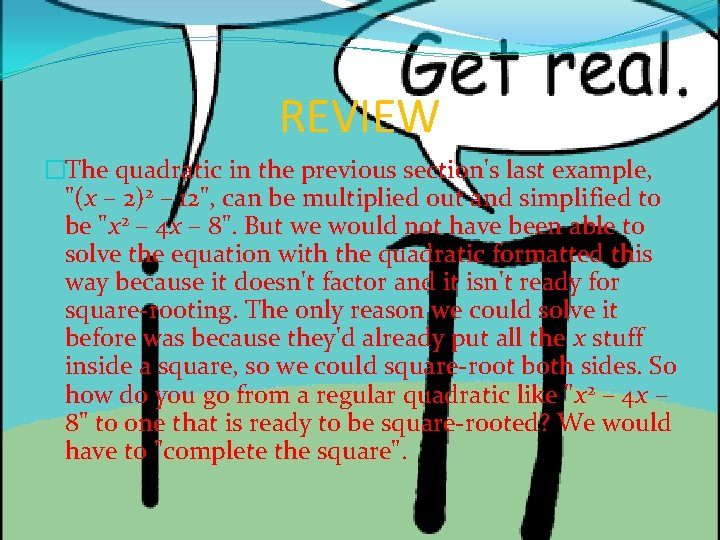REVIEW �The quadratic in the previous section's last example, "(x – 2)2 – 12", can be multiplied out and simplified to be "x 2 – 4 x – 8". But we would not have been able to solve the equation with the quadratic formatted this way because it doesn't factor and it isn't ready for square-rooting. The only reason we could solve it before was because they'd already put all the x stuff inside a square, so we could square-root both sides. So how do you go from a regular quadratic like "x 2 – 4 x – 8" to one that is ready to be square-rooted? We would have to "complete the square".�Some quadratics are fairly simple to solve because they are of the form "something-with-x squared equals some number", and then you take the square root of both sides. An example would be: �(x – 4)2 = 5 x – 4 = ± sqrt(5) x = 4 – sqrt(5) and x = 4 + sqrt(5) �Unfortunately, most quadratics don't come neatly squared like this. For your average everyday quadratic, you first have to use the technique of "completing the square" to rearrange the quadratic into the neat "(squared part) equals (a number)" format demonstrated above. For example: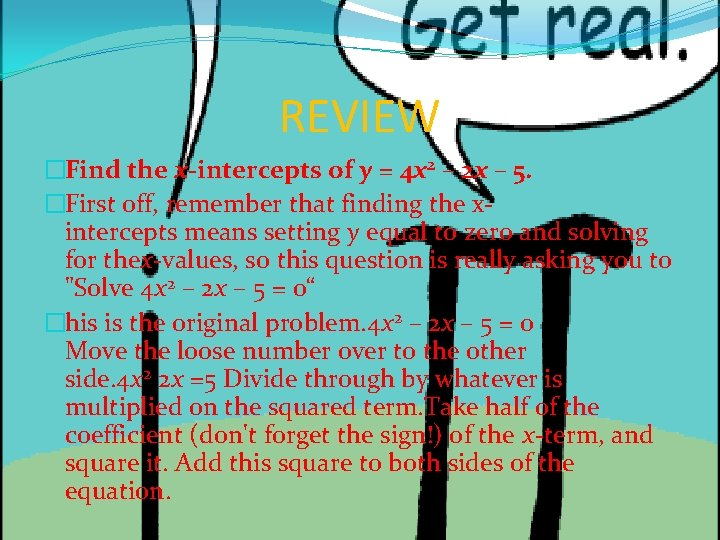REVIEW �Find the x-intercepts of y = 4 x 2 – 2 x – 5. �First off, remember that finding the xintercepts means setting y equal to zero and solving for thex-values, so this question is really asking you to "Solve 4 x 2 – 2 x – 5 = 0“ �his is the original problem. 4 x 2 – 2 x – 5 = 0 Move the loose number over to the other side. 4 x 2 2 x =5 Divide through by whatever is multiplied on the squared term. Take half of the coefficient (don't forget the sign!) of the x-term, and square it. Add this square to both sides of the equation.REVIEW �Convert the left-hand side to squared form, and simplify the right-hand side. (This is where you use that sign that you kept track of earlier. You plug it into the middle of the parenthetical part. ) �Square-root both sides, remembering the"±" on the right-hand side. Simplify as necessary. Solve for "x =". Remember that the "±" means that you have two values for x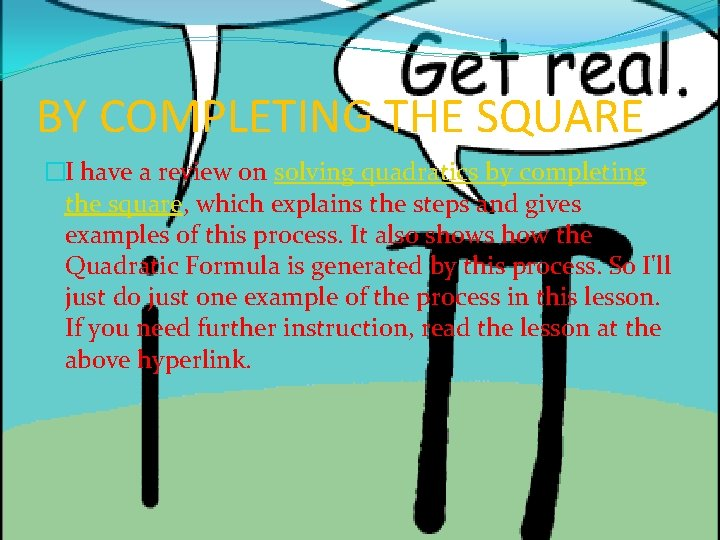BY COMPLETING THE SQUARE �I have a review on solving quadratics by completing the square, which explains the steps and gives examples of this process. It also shows how the Quadratic Formula is generated by this process. So I'll just do just one example of the process in this lesson. If you need further instruction, read the lesson at the above hyperlink.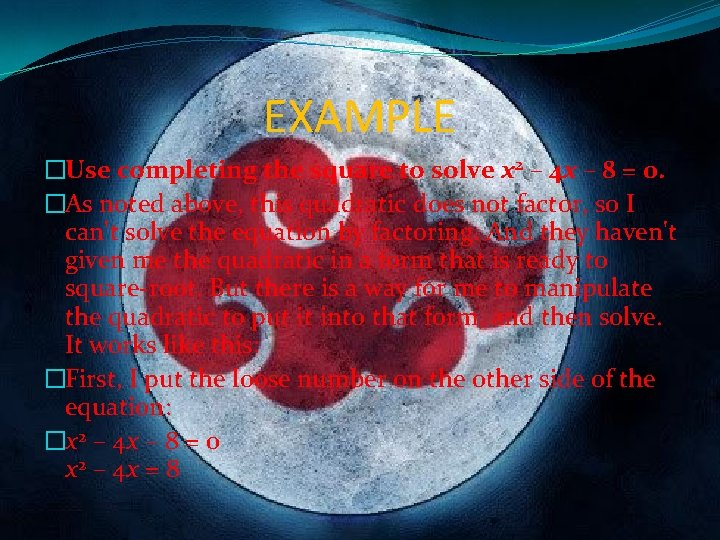EXAMPLE �Use completing the square to solve x 2 – 4 x – 8 = 0. �As noted above, this quadratic does not factor, so I can't solve the equation by factoring. And they haven't given me the quadratic in a form that is ready to square-root. But there is a way for me to manipulate the quadratic to put it into that form, and then solve. It works like this: �First, I put the loose number on the other side of the equation: �x 2 – 4 x – 8 = 0 x 2 – 4 x = 8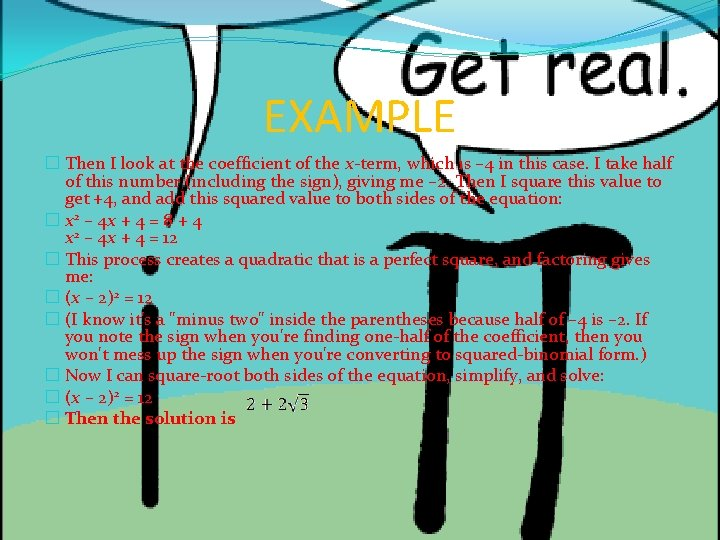EXAMPLE � Then I look at the coefficient of the x-term, which is – 4 in this case. I take half of this number (including the sign), giving me – 2. Then I square this value to get +4, and add this squared value to both sides of the equation: � x 2 – 4 x + 4 = 8 + 4 x 2 – 4 x + 4 = 12 � This process creates a quadratic that is a perfect square, and factoring gives me: � (x – 2)2 = 12 � (I know it's a "minus two" inside the parentheses because half of – 4 is – 2. If you note the sign when you're finding one-half of the coefficient, then you won't mess up the sign when you're converting to squared-binomial form. ) � Now I can square-root both sides of the equation, simplify, and solve: � (x – 2)2 = 12 � Then the solution is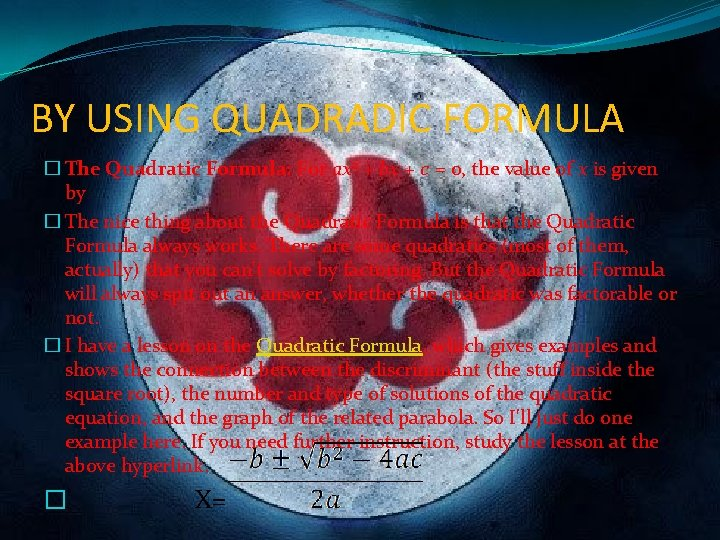BY USING QUADRADIC FORMULA � The Quadratic Formula: For ax 2 + bx + c = 0, the value of x is given by � The nice thing about the Quadratic Formula is that the Quadratic Formula always works. There are some quadratics (most of them, actually) that you can't solve by factoring. But the Quadratic Formula will always spit out an answer, whether the quadratic was factorable or not. � I have a lesson on the Quadratic Formula, which gives examples and shows the connection between the discriminant (the stuff inside the square root), the number and type of solutions of the quadratic equation, and the graph of the related parabola. So I'll just do one example here. If you need further instruction, study the lesson at the above hyperlink. � X=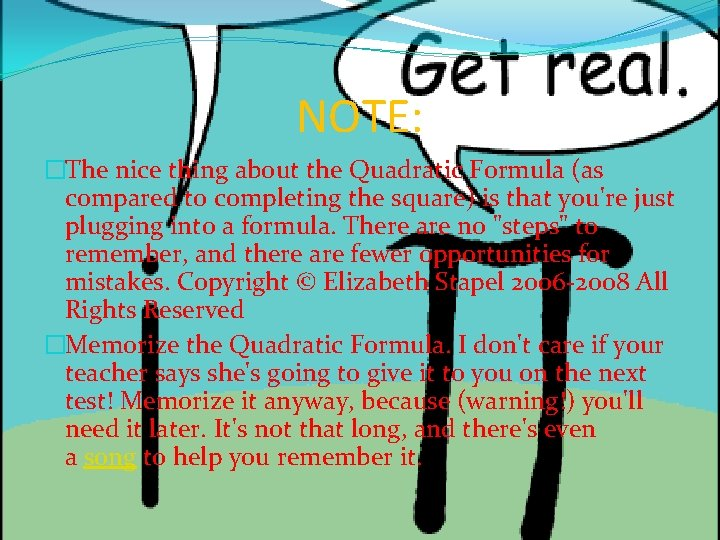NOTE: �The nice thing about the Quadratic Formula (as compared to completing the square) is that you're just plugging into a formula. There are no "steps" to remember, and there are fewer opportunities for mistakes. Copyright © Elizabeth Stapel 2006 -2008 All Rights Reserved �Memorize the Quadratic Formula. I don't care if your teacher says she's going to give it to you on the next test! Memorize it anyway, because (warning!) you'll need it later. It's not that long, and there's even a song to help you remember it.EXAMPLE �Use the Quadratic Formula to solve x 2 – 4 x – 8 = 0. �Looking at the coefficients, I see that a = 1, b = – 4, and c = – 8. I'll plug them into the Formula, and simplify. I should get the same answer as before. �Then the solution is